Next: Sound Wave Up: Gravitational Instability Previous: Gravitational Instability   Contents

## Linear Analysis

Consider a uniform gas with density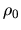and pressure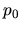without motion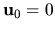. In this uniform gas distribution, we assume small perturbations. As a result, the distributions of the density, the pressure and the velocity are perturbed from the uniform ones as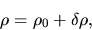(2.27)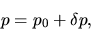(2.28)

and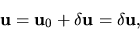(2.29)

where the amplitudes of perturbations are assumed much small, that is,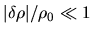,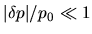and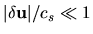. We assume the variables changes only in the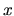-direction. In this case the basic equations for isothermal gas are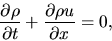(2.30)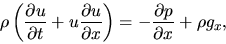(2.31)

and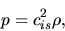(2.32)

where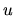and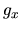represent the-component of the velocity and that of the gravity, respectively.

Using equations (2.27), (2.28), and (2.29), equation (2.30) becomes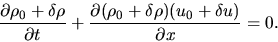(2.33)

Noticing that the amplitudes of variables with and without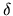are completely different, two equations are obtained from equation(2.33) as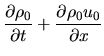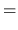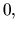(2.34)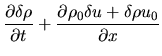(2.35)

where the above is the equation for unperturbed state and the lower describes the relation between the quantities with. Equation (2.34) is automatically satisfied by the assumption of uniform distribution. In equation (2.35) the last term is equal to zero. Equation of motion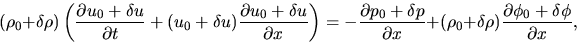(2.36)

gives the relationship between the terms containing only one variable withas follows: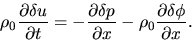(2.37)

The perturbations of pressure and density are related with each other as follows: for the isothermal gas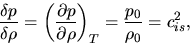(2.38)

and for isentropic gas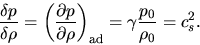(2.39)Next: Sound Wave Up: Gravitational Instability Previous: Gravitational Instability   Contents
Kohji Tomisaka 2007-07-08